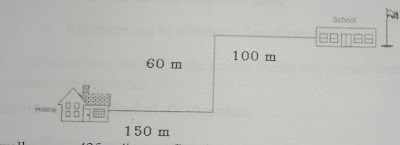# Lesson Plan of Length/Mass/Capacity

Lesson Planning of Measurement of Length, Mass and Capacity (Addition of Units of Length)

Subject Mathematics

Students` Learning Outcomes

• Add measures of length in same units with and without carrying.
• Solve real life problems involving same units of length for addition with and without carrying.

Information for Teachers

• Same units of measurement can be added, as;
• Centimetre can be added into centimetre
• Meter can be added into meter
• Kilometre can be added into kilometre.
• While teaching the lesson, the teacher should also consult text book at all steps wherein and whenever it is applicable.

Material / Resources

Writing board, chalk/marker, duster, stick, ruler, meter rode and measuring tape etc.

Introduction

• Describe a situation
• There are four baskets two of apples and two for oranges
• Ask from students how many oranges and how many apples.
• Point out that we can sum apples with apples and oranges with oranges only.
• Teacher can use copies and book alternatively.

Development

Teaching Approach

Inquiry, problem solving

 Activity 1 (Problem solving) Ask student to make pairs and each pair to measure the length of their pencils and note down in their note books. Ask them what is the total length of their pencils?10 cm + 15 cm =     Centimetre   1  0 cm +1  5 cm 2  5 cm

 Activity 2 Ask the students to add the following quantitative 40 cm  +  10 cm 15 cm  +  4 cm 35 cm  +  12 cm 5 m  +     62 m 123 km  +  76 km 100 km  +  85 km Guide the students in writing unit digit under unit digit and tens digit under tens digit and hundreds digit under hundred digit.         Centimetre,      Centimetre               4   0 cm             1  5 cm          +1  0 cm            +   4             5  0 cm            1  9 cm          Meter,            Meter           3  5 m              0  5 m     + 1 2 m          +  6  2 m        4 7 m              6  7 m   Kilometre,          Kilometre     1 2 3 km            1 0 0 km      +   7  6 km         +   8  5 km      1 9 9 km             1 8 5 km  Ask the students to solve the following sums: 100 m  +  100 m  = 57 m  +  15 m  = 16 km  +  69 km = 39 cm  +  51 cm  = 589 km  +  306 km  = 900 km  +  100 km  = 155 km  +  715 km  = 365 km  +  26 km  =

 Activity 3 (Problem solving) Ahmad`s school is shown in the following picture. Ahmad goes to school from his home and returns to home. What is the total distance he covers? Distance from Ahmad`s home to school = 150 m  +  60 m  +  100 m = 310 m Distance from Ahmad`s school to home = 150 m  +  60 m  +  100 m = 310 m Total distance = 310 m  +  310 m = 620 mNadia during her walk covers 425 km distance 1st day. Next day she covers 350 km distance. What is total distance she covers in two days?

• Distance covered on 1st day         = 425 km
• Distance covered on 2nd day       =  350 km
• Total distance covered                =   775 km

Sum up / Conclusion
• Inform to students that we have learned today how to add two or more lengths together.